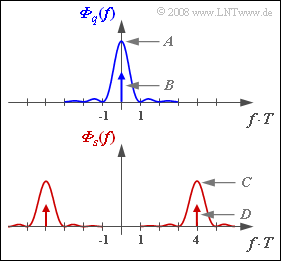# Exercise 4.7: Spectra of ASK and BPSKPower-spectral densities of  $q(t)$  and  $s(t)$  – valid for ASK and BPSK

The transmitted signals of  $\rm ASK$  ("Amplitude Shift Keying")  and  $\rm BPSK$  ("Binary Phase Shift Keying")  can both be expressed in the form

$$s(t) = q(t) · z(t),$$

where the carrier  $z(t)$  represents a harmonic oscillation with frequency  $f_{\rm T}$  and amplitude  $1$.  The carrier phase  $ϕ_{\rm T}$  is not important for the power-spectral densities considered here.

• In each case,  the source is redundancy-free,  which means that the two possible symbols are equally probable and the symbols are statistically independent of each other.
• For ASK,  the unipolar amplitude coefficients – that is:  $a_ν ∈ \{0, 1\}$  – of the source signal are
$$q(t) = \sum_{\nu = - \infty}^{+\infty}a_\nu \cdot g_q (t - \nu \cdot T)$$
while in the case of BPSK  $a_ν ∈ \{-1, +1\}$  has to be considered.

In the diagram,  the power-spectral densities  ${\it Φ}_q(f)$  and  ${\it Φ}_s(f)$  of the source signal and the transmitted signal are given,  respectively,  for an NRZ rectangular pulse  $g_q(t)$  with amplitude  $s_0 = 2 \ \rm V$  and duration  $T = 1 \ \rm µ s$.  Thus the spectral function is:

$$G_q(f) = s_0 \cdot T \cdot {\rm si}(\pi f T)\hspace{0.05cm}.$$

The constants  $A$,  $B$,  $C$  and  $D$  for the  $\rm ASK$  and  $\rm BPSK$ modulation methods are to be determined.

Notes:

• The exercise belongs to the chapter  Linear Digital Modulation.
• Reference is also made to the chapter  Basics of Coded Transmission  in the book  "Digital Signal Transmission".
• The powers are to be specified in  $\rm V^2$;  they thus refer to the reference resistance  $R = 1 \ \rm \Omega$.

### Questions

1

Which values result with ASK for the parameters  $A = {\it Φ}_q(f = 0)$  and  $B$  $($Dirac weight at  $f = 0)$?

 $A \ = \$ $\ \cdot 10^{-6} \ \rm V^2/Hz$ $B \ = \$ $\ \rm V^2$

2

Determine the parameters  $C = {\it Φ}_s(f = f_{\rm T})$  and  $D$  $($Dirac weight at $f = f_{\rm T})$ for the ASK transmitted signal.

 $C \ = \$ $\ \cdot 10^{-6} \ \rm V^2/Hz$ $D \ = \$ $\ \rm V^2$

3

What are the values for parameters  $A$  and  $B$  for BPSK?

 $A \ = \$ $\ \cdot 10^{-6} \ \rm V^2/Hz$ $B \ = \$ $\ \rm V^2$

4

What are the values of parameters  $C$  and  $D$  for BPSK?

 $C \ = \$ $\ \cdot 10^{-6} \ \rm V^2/Hz$ $D \ = \$ $\ \rm V^2$

5

Which statements are always true for BPSK even if  $g_q(t)$  is not an NRZ rectangular pulse?

 The continuous part of  ${\it Φ}_q(f)$  is equal in form to  $|G_q(f)|^2$. ${\it Φ}_q(f)$  contains a single Dirac delta line at ASK  for BPSK $($at $f = 0)$. ${\it Φ}_q(f)$ contains a single Dirac delta line at BPSK  for BPSK $($at $f = 0)$.

### Solution

#### Solution

(1)  The DC component of the unipolar redundancy-free source signal is  $m_q = s_0/2$.  Thus,  the Dirac weight is  $B = m_q^2 = s_0^2/4\hspace{0.15cm}\underline{ = 1 \ \rm V^2}$.

• Without this DC component,  the stochastic signal  $q(t) - m_q ∈ \{+s_0/2, -s_0/2\}$ would be obtained.
• This DC-free signal has the continuous PSD component  $(s_0/2)^2 · T · {\rm sinc}^2(fT)$.
• From this,  the value we are looking for at frequency  $f = 0$  can be determined:
$$A = \frac {s_0^2 \cdot T }{4} = \frac {(2\,{\rm V})^2 \cdot 10^{-6} \,{\rm s}}{4}\hspace{0.15cm}\underline {= 10^{-6} \,{\rm V^{2}/Hz}}.$$

(2)  The spectrum  $Z(f)$  of a cosine signal  $z(t)$  consists of two Dirac delta functions at  $\pm f_{\rm T}$,  each with weight  $1/2$.

• The power-spectral density  ${\it Φ}_z(f)$  also consists of the two Dirac delta functions,  but now with respective weights  $1/4$.
• The convolution  ${\it Φ}_q(f) ∗ {\it Φ}_z(f)$  gives the power-spectral density  ${\it Φ}_s(f)$  of the transmitted signal.  It follows that:
$$C = {A}/{4} \hspace{0.15cm}\underline { = 0.25 \cdot 10^{-6} \,{\rm V^{2}/Hz}},\hspace{0.2cm}D = {B}/{4}\hspace{0.15cm}\underline { = 0.25 \,{\rm V^{2}}}.$$

Note:   The power per bit is obtained as the integral over  ${\it Φ}_s(f)$:

$$P_{\rm S} = \int_{ - \infty }^\infty \hspace{-0.3cm} {{\it \Phi}_s(f)}\hspace{0.1cm} {\rm d}f = 2 \cdot \int_{ 0 }^\infty \hspace{-0.3cm} {\left [ C \cdot {\rm sinc}^2(f T) + D \cdot \delta (f - f_{\rm T}]\right ]}\hspace{0.1cm} {\rm d}f= 2 \cdot \left [ \frac{C}{T} + D \right ] = 2 \cdot \left [ \frac{0.25 \cdot 10^{-6} \,{\rm V^{2}/Hz}}{10^{-6} \,{\rm s}} + 0.25 \,{\rm V^{2}} \right ] \hspace{0.15cm}\underline {= 1 \,{\rm V^{2}}}.$$

(3)  For BPSK, the source signal  $q(t)$  is to be bipolar.

• Therefore,  the Dirac delta line is missing in the power-spectral density  ⇒   $\underline{B = 0}$.
• The continuous PSD component is four times larger than with ASK:
$$A = {s_0^2 \cdot T }\hspace{0.15cm}\underline { = 4 \cdot 10^{-6} \,{\rm V^{2}/Hz}}.$$

(4)  For the PSD parameters of the BPSK transmitted signal,  the following applies analogously to the ASK:

$$C = \frac {A}{4}\hspace{0.15cm}\underline { = 10^{-6} \,{\rm V^{2}/Hz}},\hspace{0.2cm}D = \frac {B}{4} \hspace{0.15cm}\underline {= 0}.$$

(5)  Only the  first statement  is correct:

• For BPSK,  ${\it Φ}_q(f)$  does not contain a single Dirac delta line even if  $g_q(t)$  deviates from the rectangular form  (assuming equally probable symbols).
• In contrast, the unipolar ASK source signal contains infinitely many Dirac delta lines at all multiples of  $1/T$.
• For more information on this topic, see the page  "ACF and PSD for unipolar binary signals" in the book "Digital Signal Transmission".